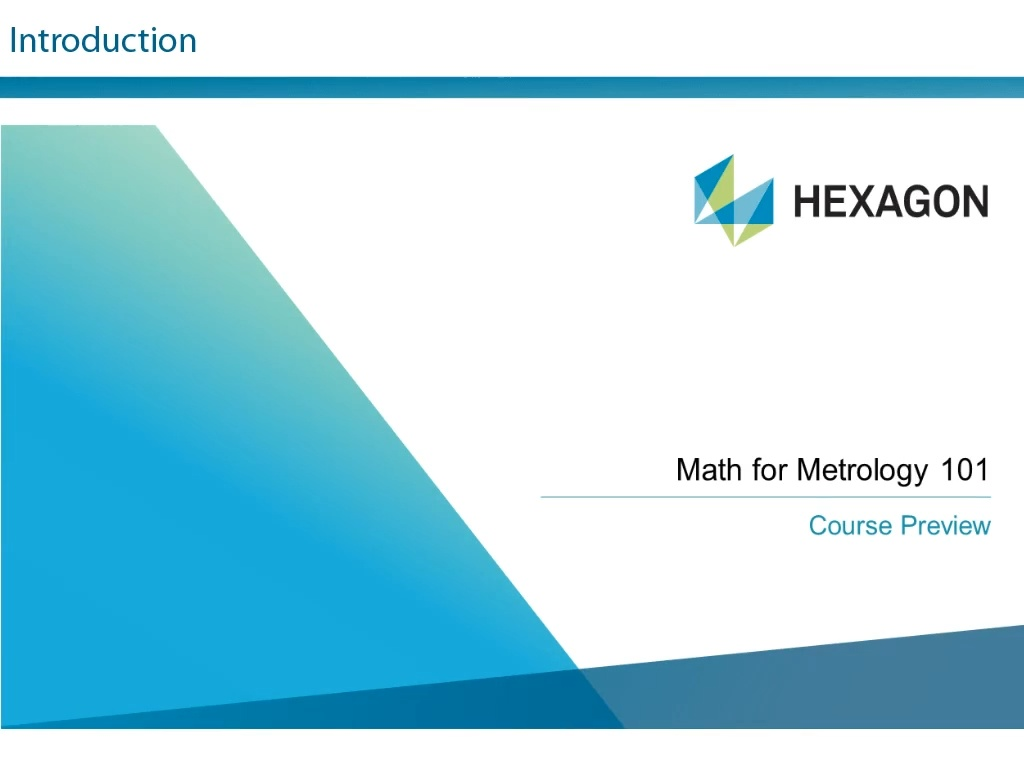You are not logged in.Language:Math for Metrology 101 (11050-11-20)

\$49.00 (USD)

This eLearning course introduces the student to the math concepts needed to succeed. The course teaches concepts such as integers, using parentheses, rounding, units of length, coordinate systems, and much more.Estimated Length: 1 hour(s) and 30 minute(s)

## Lessons

Name View Schedule1. Math for Metrology 101 -2. Online Class Survey -

## Objectives

• Understand Integers, Reals, Fractions and Decimal numbers
• Understand the use of parentheses in equations
• Understand and apply Square and Square Roots
• Round numbers Up and Down and understand the residual error
• Understand units of Length, Angle and Temperature
• Understand Cartesian and Polar coordinate systems
• Understand Dimension Nominals, Tolerances and Deviation directions